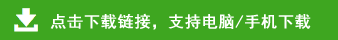# 一年级数学下册模拟检测卷

一年级数学下册模拟检测卷

37+9=     47+8=     64-9=

73-20=    44+40=    71-10=

48+30+10=

62-20+30=

84-50+20=

55-7+40=

21+30-9=

60+（20-7）=

100-20-30=

1、一个数由3个十和5个一组成，这个数是（     ），读作：（      ）和它相邻的两个数是(    )和（    ）。

2、一张100元的人民币，可以换成（      ）张50元，或者还能换成（     ）张10元的。